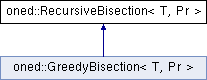# oned::RecursiveBisection< T, Pr > Class Template Reference

recursive 1D partitioning algorithm which partition an array in two parts of similiar load and allocates half the processor in each part. More...

`#include <oned/rec_bisect.hpp>`

Inheritance diagram for oned::RecursiveBisection< T, Pr >:## Detailed Description

### template<typename T, typename Pr> class oned::RecursiveBisection< T, Pr >

recursive 1D partitioning algorithm which partition an array in two parts of similiar load and allocates half the processor in each part.

Parameters:
 T data type of the Prefix Sum Array Pr type of the Prefix Sum Array

List of all members.

## Static Public Member Functions

static double calculateDiff (const Pr &prefixSum, int low, int high, int leftProc, int rightProc, int candidate)
static int findEvenCut (const Pr &prefixSum, int low, int high, int leftProc, int rightProc)
static T rec_bisection (int procCount, const Pr &prefixSumArray, int length, int *cutIndexes, T max)

## Static Private Member Functions

static T rec_bisection_internal (int numProc, int low, int high, const Pr &prefixSum, int *cutPoints)

## Static Private Attributes

static const bool debugFEC = false
static const bool debugRB = false

## Member Function Documentation

template<typename T , typename Pr >
 double oned::RecursiveBisection< T, Pr >::calculateDiff ( const Pr & prefixSum, int low, int high, int leftProc, int rightProc, int candidate ) ` [static]`
template<typename T , typename Pr >
 int oned::RecursiveBisection< T, Pr >::findEvenCut ( const Pr & prefixSum, int low, int high, int leftProc, int rightProc ) ` [static]`

Finds the best cut point of a given array by calculating Wtot*(leftProc)/(leftProc+rightProc) and then decides whether to cut from left or right. left and low index values are included to calculation. prefixsum = 0.

Parameters:
 prefixSum 1D prefixsum array low lower bound high upper bound leftProc number of processor on left side rightProc number of processor on right side
Returns:
cut index (corresponds to the last element of leftProc and one earlier than first element of rightProc)
template<typename T , typename Pr >
 T oned::RecursiveBisection< T, Pr >::rec_bisection ( int procCount, const Pr & prefixSumArray, int length, int * cutIndexes, T max ) ` [static]`
Parameters:
 [in] procCount number of processors [in] prefixSumArray Prefix sum array that always starts with value 0 in the 0th index [in] length is the length of prefixSumArray [out] cutIndexes cut index points [in] max maximum load of a given array. If unknown, use -1. (ignored in this function.)
Returns:
 T oned::RecursiveBisection< T, Pr >::rec_bisection_internal ( int numProc, int low, int high, const Pr & prefixSum, int * cutPoints ) ` [static, private]`
 const bool oned::RecursiveBisection< T, Pr >::debugFEC = false` [static, private]`
 const bool oned::RecursiveBisection< T, Pr >::debugRB = false` [static, private]`